MATLAB Function Referencebvpinit

Form the initial guess for `bvp4c`

Syntax

• ```solinit = bvpinit(x,yinit)
solinit = bvpinit(x,yinit,parameters)
solinit = bvpinit(sol,[anew bnew])
solinit = bvpinit(sol,[anew bnew],parameters)
```

Description

```solinit = bvpinit(x,yinit) ``` forms the initial guess for the boundary value problem solver `bvp4c`.

`x` is a vector that specifies an initial mesh. If you want to solve the boundary value problem (BVP) on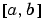, then specify `x(1)` as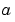and `x(end)` as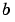. The function `bvp4c` adapts this mesh to the solution, so a guess like `x = linspace(a,b,10)` often suffices. However, in difficult cases, you should place mesh points where the solution changes rapidly. The entries of `x` must be in

• Increasing order if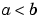• Decreasing order if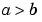For two-point boundary value problems, the entries of `x` must be distinct. That is, if, the entries must satisfy `x(1)` < `x(2)` < ... < `x(end)`. If, the entries must satisfy `x(1)` > `x(2)` > ... > `x(end)`

For multipoint boundary value problem, you can specify the points inat which the boundary conditions apply, other than the endpoints a and b, by repeating their entries in `x`. For example, if you set

• ```x = [0, 0.5, 1, 1,  1.5, 2];
```

the boundary conditions apply at three points: the endpoints `0` and `2`, and the repeated entry 1. In general, repeated entries represent boundary points between regions in. In the preceding example, the repeated entry 1 divides the interval `[0,2]` into two regions: `[0,1]` and `[1,2]`.

`yinit` is a guess for the solution. It can be either a vector, or a function:

• Vector - For each component of the solution, `bvpinit` replicates the corresponding element of the vector as a constant guess across all mesh points. That is, `yinit(i)` is a constant guess for the `i`th component `yinit(i,:)` of the solution at all the mesh points in `x`.
• Function - For a given mesh point, the guess function must return a vector whose elements are guesses for the corresponding components of the solution. The function must be of the form
• ```y = guess(x)
```
• where `x` is a mesh point and `y` is a vector whose length is the same as the number of components in the solution. For example, if the guess function is an M-file function, `bvpinit` calls

• ``````y(:,j) = guess(x(j))
``````

at each mesh point.

For multipoint boundary value problems, the guess function must be of the form

• ```y = guess(x, k)
```

where `y` an initial guess for the solution at `x` in region `k`. The function must accept the input argument `k`, which is provided for flexibility in writing the guess function. However, the function is not required to use `k`.

```solinit = bvpinit(x,yinit,parameters) ``` indicates that the boundary value problem involves unknown parameters. Use the vector `parameters` to provide a guess for all unknown parameters.

`solinit` is a structure with the following fields. The structure can have any name, but the fields must be named `x`, `y`, and `parameters`.

 `x` Ordered nodes of the initial mesh. `y` Initial guess for the solution with `solinit.y(:,i)` a guess for the solution at the node `solinit.x(i)`. `parameters` Optional. `A `vector that provides an initial guess for unknown parameters.

```solinit = bvpinit(sol,[anew bnew]) ``` forms an initial guess on the interval `[anew bnew]` from a solution `sol` on an interval. The new interval must be larger than the previous one, so either `anew <= `a` < `b `<= bnew` or `anew >= `a` > `b `>= bnew`. The solution `sol` is extrapolated to the new interval. If `sol` contains `parameters`, they are copied to `solinit`.

```solinit = bvpinit(sol,[anew bnew],parameters) ``` forms `solinit` as described above, but uses `parameters` as a guess for unknown parameters in `solinit`.

See Also

`@` (`function_handle`), `bvp4c`, `bvpget`, `bvpset`, `deval`

© 1994-2005 The MathWorks, Inc.